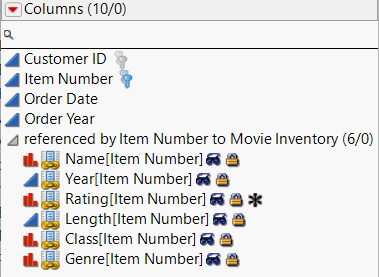Publication date: 04/12/2021

## Virtually Join Data Tables

Virtual Join links a main data table to one or more auxiliary data tables. The feature enables the main data table to access data from the auxiliary data tables without physically joining the tables. See Virtually Join Data Tables in Using JMP.

The following example shows how to virtually join data tables and create distributions from the joined data:

`dt1 = Open( "\$SAMPLE_DATA/Pizza Profiles.jmp" );`
`dt1:ID << Set Property( "Link ID", 1 );// add Link ID and turn it on`
` `
`dt2 = Open( "\$SAMPLE_DATA/Pizza Responses.jmp" );`
` `

// add Link Reference to the Choice1, Choice2, and Choice columns

`dt2:Choice1 << Set Property( "Link Reference", Reference Table( "\$SAMPLE_DATA/Pizza Profiles.jmp" ) );`
`dt2:Choice2 << Set Property( "Link Reference", Reference Table( "\$SAMPLE_DATA/Pizza Profiles.jmp" ) );`
`dt2:Choice << Set Property( "Link Reference", Reference Table( "\$SAMPLE_DATA/Pizza Profiles.jmp" ) );`
` `
`obj = dt2 << Distribution( // create a distribution of Crust[Choice1]`
`	Weight( :Subject ),`
`	Nominal Distribution(`
`		Column(`
`			Referenced Column(`
`				"Crust[Choice1]", // specify the joined column name`
`				Reference( Column( :Choice1 ), Reference( Column( :Crust ) ) )`
`			)`
`		)`
`	),`
`	Nominal Distribution( Column( :Choice1 ) )`
`);`

For another example of virtual join, search the Help > Scripting Index for “virtual join”.

#### Specifying Linked Column Names

The Columns panel in a data table shows you the names of the linked columns. For example, open the Movie Inventory.jmp and Movie Rentals.jmp sample data tables to see the linked columns shown in Figure 9.12.

The column name of the source column is followed by the name of the referencing column in brackets. For example, Figure 9.12 shows the Name source column followed by the referencing column [Item Number] in brackets.

Figure 9.12 Linked Columns in Movie Rentals.jmpYou change the name of the virtually linked column name with Set Title. For example, the following script changes the name of the virtually linked column Rating[Item Number] to Movie Rating in the Distribution report.

`dt1 = Open( "\$SAMPLE_DATA/Movie Inventory.jmp" );`
`dt2 = Open( "\$SAMPLE_DATA/Movie Rentals.jmp" );`
`dt2 << Distribution(`
`	Nominal Distribution(`
`		Column(`
`			Referenced Column( // specify the joined column name`
`				"Rating[Item Number]",`
`				Reference( Column( :Item Number ), Reference( Column( :Rating ) ) )`
`			)`
`		)`
`	),`
`	SendToReport( Dispatch( {}, "Rating[Item Number]", OutlineBox,`
`		{Set Title( "Movie Rating" )} ) ) /* change the name of the`
`		virtually linked column */`
`);`

Note that running the script automatically opens the referenced table (the one with the Link ID property), just like running any other platform script, before the referenced table is opened.

You can also specify that the referenced column name be used in linked columns. This option lets you define a shorter column name (for example, Rating instead of Rating[Item Number]). Include the Use Linked Column Name option:

`dt1 = Open( "\$SAMPLE_DATA/Movie Inventory.jmp" );`
`dt2 = Open( "\$SAMPLE_DATA/Movie Rentals.jmp" );`
` `
`Column( dt2, "Item Number" ) << Set Property(`
`	"Link Reference",`
`	{Reference Table( "Movie Inventory.jmp" ), Options( "Use Linked Column Name"( 1 ) )}`
`);`
`dt2 << Distribution(`
`	Nominal Distribution(`
`		Column(`
`			Referenced Column( // specify the joined column name`
`				"Rating",`
`				Reference( Column( :Item Number ), Reference( Column( :Rating ) ) )`
`			)`
`		)`
`	)`
`);`

#### Merging Referenced Data into One Data Table

To share the data from virtually joined data tables with another user, you might want to merge the data so that the data tables are permanently joined. This option is helpful because you provide one data table, not the main and auxiliary data tables.

When you merge referenced data, the data are replicated from the auxiliary data table to the main data table. When you save the main data table, the actual data are also saved.

The following example shows how to merge two referenced columns:

`dt1 = Open( "\$SAMPLE_DATA/Pizza Profiles.jmp" );`
`dt2 = Open( "\$SAMPLE_DATA/Pizza Responses.jmp" );`
` `
`dt1:ID << Set Property( "Link ID", 1 );`
` `
`dt2:Choice << Set Property( "Link Reference", Reference Table( dt1 ) );`
`dt2:Choice1 << Set Property( "Link Reference", Reference Table( dt1 ) );`
`dt2:Choice2 << Set Property( "Link Reference", Reference Table( dt1 ) );`
` `

// select the columns to merge

`dt2 << Select Columns( :Choice1, :Choice2 );`
` `
`dt2 << Merge Referenced Data();`

/* linked data from the referenced columns are now embedded in

Pizza Responses.jmp */

Note: If you don’t select the columns to merge, all columns are merged.

Want more information? Have questions? Get answers in the JMP User Community (community.jmp.com).